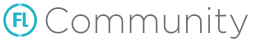I have a utility function to randomize array with int values:

``````public static function generateNewRandomizedArray(pArr:Array<Int> ):Array<Int>
{
var ret_arr:Array<Int>  = new Array<Int>() ;
var j=0;
var k = pArr.length ;
for ( i in 0... k  )
{
j= Std.int(Math.random() * pArr.length) ;
ret_arr[i]= pArr[j] ;
pArr.splice(j,1);
}

return ret_arr ;

}
``````

Is it possible to make it accept any type of value, say float, movieclip, point etc? I know how to use Dynamic to do this, but is it possible to do it through function overloading? I tried to use two functions with different signatures but got compilation error. In fact, I am not able to use Dynamic type too, as it shows compilation error while I am trying to return back array with dynamic type.

You can’t overload a function like in C#

In your case you could use “Type parameters” https://haxe.org/manual/type-system-type-parameters.html

Bonus track:

``````	/**
* Shuffles the array.
* Not in place since there is no native "swap" function.
* @param array the array to be swapped
*/
public static function shuffleArray<T>(array:Array<T>) {
// Bad implementation of Fisher and Yates' shuffle algorithm
var aux:T;
var random:Int;
var i = array.length;
while (i-- > 1) {
random = Std.random(i + 1);
aux = array[random];
array[random] = array[i];
array[i] = aux;
}
}
``````

I rembered I have a function that does a random

1 Like

This is not working for me. Shows compiler error, saying:

``````public static function generateNewRandomizedArray(pArr:Array<T> ):Array<T>
{
var ret_arr:Array<T>  = new Array<T>() ;
var j=0;
var k = pArr.length ;
for ( i in 0... k  )
{
j= Std.int(Math.random() * pArr.length) ;
ret_arr[i]= pArr[j] ;
pArr.splice(j,1);
}

return ret_arr ;

}``````

You didn’t declare the type parameter, i.e. there’s a `<T>` missing after the function name:

``````public static function generateNewRandomizedArray<T>(pArr:Array<T> ):Array<T>
``````
1 Like